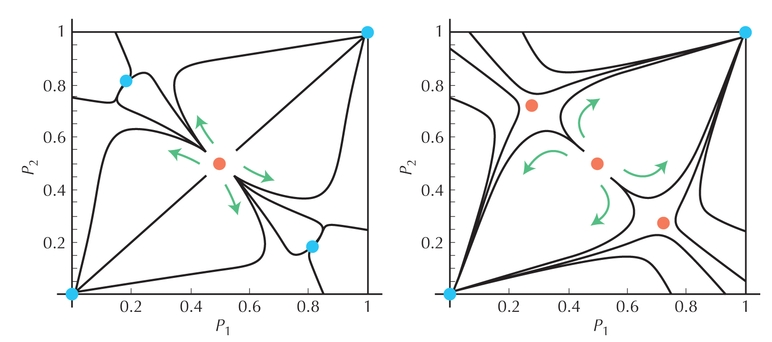Figure P18.6. Evolution of a chromosomal arrangement in two demes, under gene flow and selection against heterozygotes. Allele frequencies in two demes (p1, p2) are plotted on the two axes, with the population starting at various points. (Red) Unstable equilibria; (blue) stable equilibria. The left panel is for M = m/s = 0.15. There are two stable equilibria with different arrangements common in different demes (top left, bottom right), separated by an unstable equilibrium at p1 = p2 = 1/2. The right panel is for a higher rate of gene flow (M = m/s = 0.2). Now, the two asymmetrical equilibria exist, but are unstable (red dots at top left, bottom right): One or the other arrangement will fix in both demes.